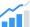# SpGEMM Targeting RISC-V Vector Processors (Barcelona Supercomputing Center)A new technical paper titled “Optimization of SpGEMM with Risc-V vector instructions” was published (preprint) by researchers at the Barcelona Supercomputing Center.

Abstract
“The Sparse GEneral Matrix-Matrix multiplication (SpGEMM) C=A×B is a fundamental routine extensively used in domains like machine learning or graph analytics. Despite its relevance, the efficient execution of SpGEMM on vector architectures is a relatively unexplored topic. The most recent algorithm to run SpGEMM on these architectures is based on the SParse Accumulator (SPA) approach, and it is relatively efficient for sparse matrices featuring several tens of non-zero coefficients per column as it computes C columns one by one. However, when dealing with matrices containing just a few non-zero coefficients per column, the state-of-the-art algorithm is not able to fully exploit long vector architectures when computing the SpGEMM kernel. To overcome this issue we propose the SPA paRallel with Sorting (SPARS) algorithm, which computes in parallel several C columns among other optimizations, and the HASH algorithm, which uses dynamically sized hash tables to store intermediate output values. To combine the efficiency of SPA for relatively dense matrix blocks with the high performance that SPARS and HASH deliver for very sparse matrix blocks we propose H-SPA(t) and H-HASH(t), which dynamically switch between different algorithms. H-SPA(t) and H-HASH(t) obtain 1.24× and 1.57× average speed-ups with respect to SPA respectively, over a set of 40 sparse matrices obtained from the SuiteSparse Matrix Collection. For the 22 most sparse matrices, H-SPA(t) and H-HASH(t) deliver 1.42× and 1.99× average speed-ups respectively.”

Find the technical paper here. Published March 2023.

Fèvre, V. L., & Casas, M. (2023). Optimization of SpGEMM with Risc-V vector instructions. arXiv preprint arXiv:2303.02471